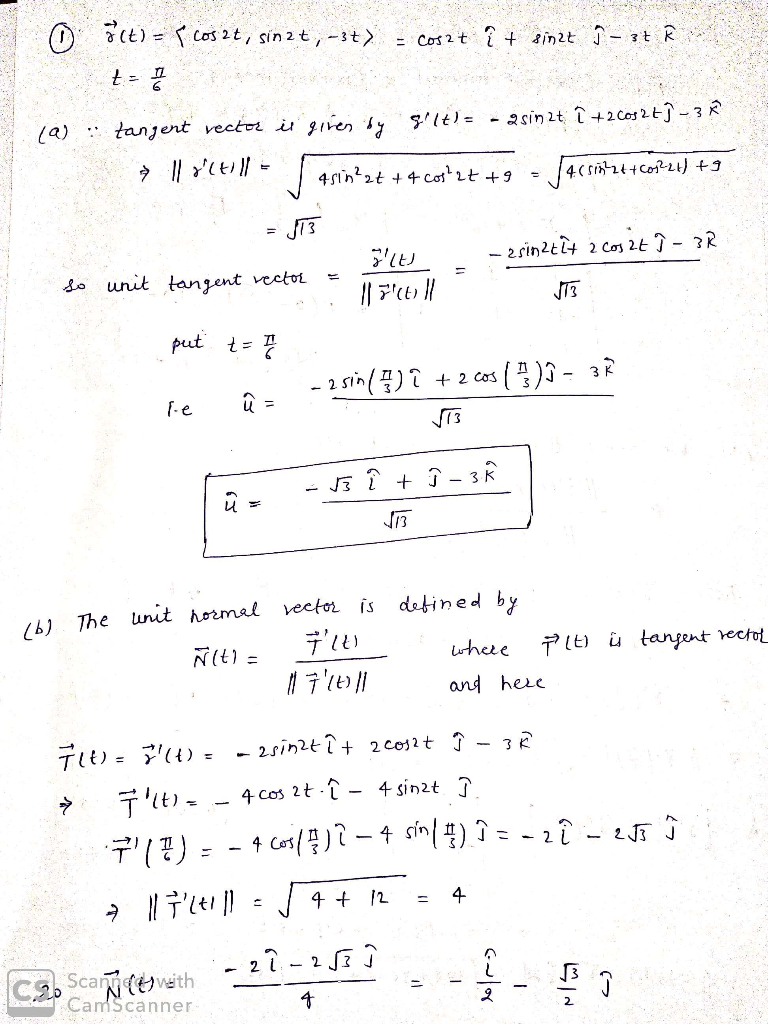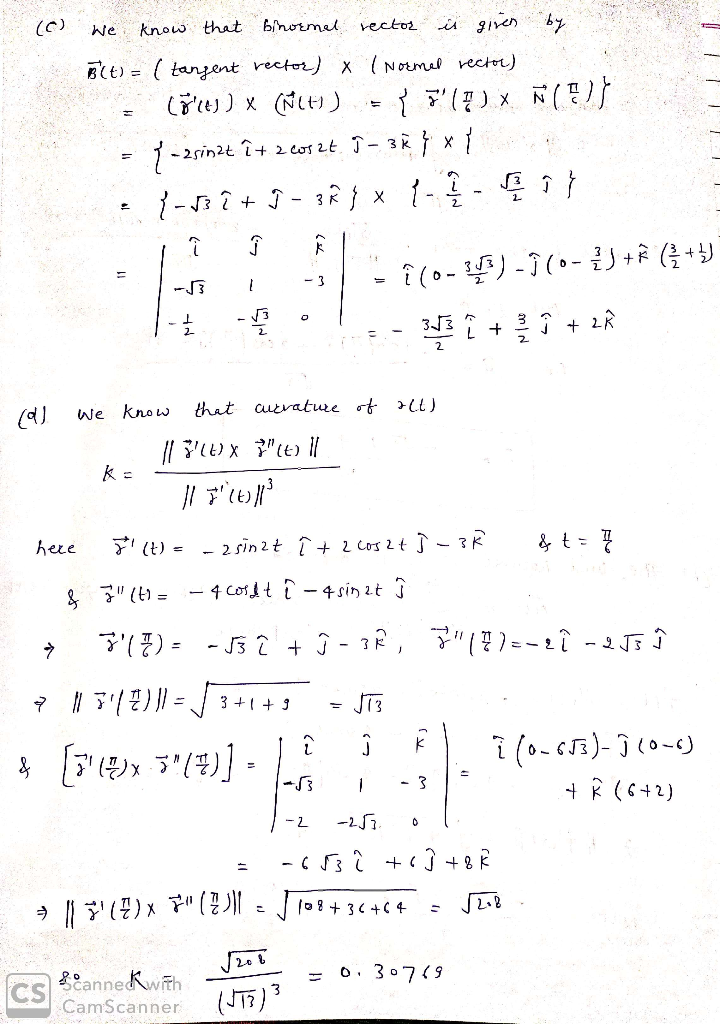In: Math

# Consider the helix r(t)=(cos(2t),sin(2t),−3t)r(t)=(cos(2t),sin(2t),−3t). Compute, at t=π/6 A. The unit tangent vector T=T= ( , ,...

Consider the helix r(t)=(cos(2t),sin(2t),−3t)r(t)=(cos(2t),sin(2t),−3t). Compute, at t=π/6

A. The unit tangent vector T=T= ( , , )

B. The unit normal vector N=N= ( , , )

C. The unit binormal vector B=B= ( , , )

D. The curvature κ=κ=

## Solutions

##### Expert Solution## Related Solutions

##### Consider the vector function given below. r(t) = 2t, 3 cos(t), 3 sin(t) (a) Find the...
Consider the vector function given below. r(t) = 2t, 3 cos(t), 3 sin(t) (a) Find the unit tangent and unit normal vectors T(t) and N(t). T(t)   =    N(t)   =    (b) Use this formula to find the curvature. κ(t) =
##### Consider the spiral path x(t) = (cos^2t,sin^2t,t) for 0 ≤ t ≤ π/2. Evaluate the integral...
Consider the spiral path x(t) = (cos^2t,sin^2t,t) for 0 ≤ t ≤ π/2. Evaluate the integral x dx−y dy + z^2 dz
##### Consider the following vector function. r(t) =<3t, 1/2 t2, t2> (a) Find the unit tangent and...
Consider the following vector function. r(t) =<3t, 1/2 t2, t2> (a) Find the unit tangent and unit normal vectors T(t) and N(t). (b). Find the curvature k(t).
##### 3. Consider the parametric curve x = sin 2t, y = − cos 2t for −π/4...
3. Consider the parametric curve x = sin 2t, y = − cos 2t for −π/4 ≤ t ≤ π/4. (a) (2 pts) Find the Cartesian form of the curve. (b) (3 pts) Sketch the curve. Label the starting point and ending point, and draw an arrow on the curve to indicate the direction of travel. (c) (5 pts) Find an equation for the curve’s tangent line at the point √2/2, −√2/2 .
##### 3sin(t)+3sin(t)sin(2t)=3cos(t)-cos(3t). I'm trying to solve for t.
3sin(t)+3sin(t)sin(2t)=3cos(t)-cos(3t). I'm trying to solve for t.
##### (1 point) For the given position vectors r(t)r(t) compute the unit tangent vector T(t)T(t) for the...
(1 point) For the given position vectors r(t)r(t) compute the unit tangent vector T(t)T(t) for the given value of tt . A) Let r(t)=〈cos5t,sin5t〉 Then T(π4)〈 B) Let r(t)=〈t^2,t^3〉 Then T(4)=〈 C) Let r(t)=e^(5t)i+e^(−4t)j+tk Then T(−5)=
##### compute the unit tangent vector T and the principal normal unit vector N of the space...
compute the unit tangent vector T and the principal normal unit vector N of the space curve R(t)=<2t, t^2, 1/3t^3> at the point when t=1. Then find its length over the domain [0,2]
##### Given r(t) = <2 cos(t), 2 sin(t), 2t>. • What is the arc length of r(t)...
Given r(t) = <2 cos(t), 2 sin(t), 2t>. • What is the arc length of r(t) from t = 0 to t = 5. SET UP integral but DO NOT evaluate • What is the curvature κ(t)?
##### Let r(t) = (cos(πt), sin(πt), 3t). Calculate r'(t), T(t) and evaluate T(1).
Let r(t) = (cos(πt), sin(πt), 3t). Calculate r'(t), T(t) and evaluate T(1).
##### For this parametrized curve: x = e^(2t) sin t , y = cos(4t) find tangent line...
For this parametrized curve: x = e^(2t) sin t , y = cos(4t) find tangent line to curve when t=1